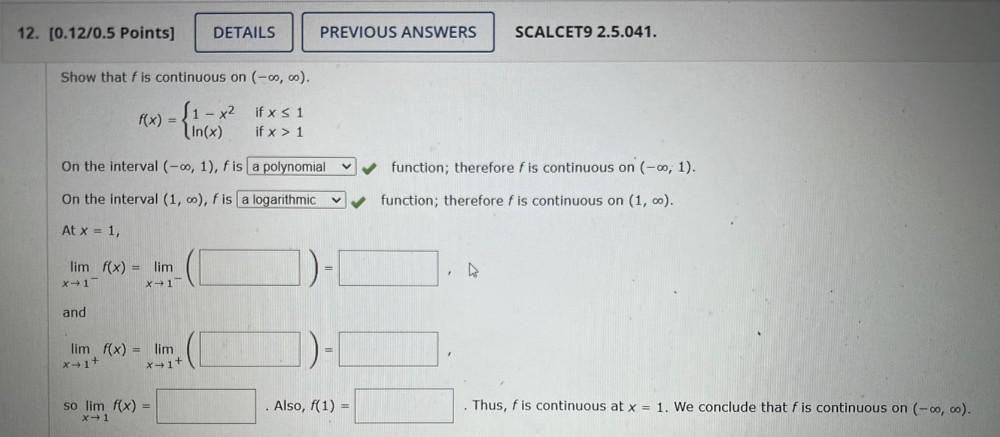Question:

# 12. (0.12/0.5 Points] DETAILS PREVIOUS ANSWERS SCALCET9 2.5.041. Show that f is continuous on (-00,00). f(x) - { ſ1 - x2 if x S12. (0.12/0.5 Points] DETAILS PREVIOUS ANSWERS SCALCET9 2.5.041. Show that f is continuous on (-00,00). f(x) - { ſ1 - x2 if x S 1 In(x) if x > 1 On the interval (-0, 1), f is a polynomial function; therefore f is continuous on (-00, 1). On the interval (1, 0), f is a logarithmic function; therefore f is continuous on (1, 0). At x = 1, lim f(x) = lim x-1 x-1 D- and lim f(x) = lim 1)- xtit x+1+ so lim f(x) = x1 Also, f(1) = Thus, f is continuous at x = 1. We conclude that f is continuous on (-0,co).